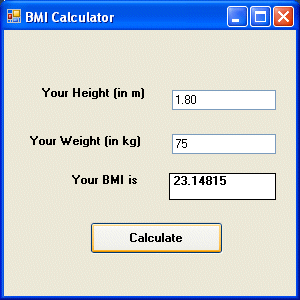># Lesson 7 : Mathematical Operations

Computer can perform mathematical calculations much faster than human beings. However, computer itself will not be able to perform any mathematical calculations without receiving instructions from the user. In VB2010, we can write code to instruct the computer to perform mathematical calculations such as addition, subtraction, multiplication, division and other kinds of arithmetic operations. In order for VB2010 to carry out arithmetic calculations, we need to write code that involve the use of various arithmetic operators. The VB2010 arithmetic operators are very similar to the normal arithmetic operators, only with slight variations. The plus and minus operators are the same while the multiplication operator use the * symbol and the division operator use the / symbol. The list of VB2010 arithmetic operators are shown in table 7.1  below:

#### Table 7.1 Arithmetic Operators

Operator Mathematical Function Example
-  Subtraction  10-4=6
^  Exponential  3^2=9
*  Multiplication  5*6=30
/  Division  21/7=3
Mod  Modulus(returns the remainder of an integer division)  15 Mod 4=3
\  Integer Division(discards the decimal places)  19/4=4

#### Example 7.1

In this program, you need to insert two Textboxes, four labels and one button. Click the button and key in the code as shown below. Note how the various arithmetic operators are being used. When you run the program, it will perform the four basic arithmetic operations and display the results on the four labels.

```Dim num1, num2, difference, product, quotient As Single
num1 = TextBox1.Text
num2 = TextBox2.Text
sum=num1+num2
difference=num1-num2
product = num1 * num2
quotient=num1/num2
Label1.Text=sum
Label2.Text=difference
Label3.Text = product
Label4.Text = quotient
```

#### Example 7.2 Pythagoras Theorem

The program can use Pythagoras Theorem to calculate the length of hypotenuse  c given the length of the adjacent side a and the opposite side b. In case you have forgotten the formula for the Pythagoras Theorem, it is written as

`			c^2=a^2+b^2`
```				The Code
Private Sub Button1_Click(ByVal sender As System.Object, ByVal e As System.EventArgs) Handles Button1.Click
Dim a, b, c As Single
a = TextBox1.Text
b = TextBox2.Text
c=(a^2+b^2)^(1/2)
Label3.Text=c
End Sub
```

#### Example 7.3: BMI Calculator

A lot of people are obese now and it could affect their health seriously . Obesity has proven by the medical experts to be a one of the main factors that brings many adverse medical problems, including the the heart disease.   If your BMI is more than 30, you are considered obese. You can refer to the following range of BMI values for your weight status.

• Underweight = <18.5
• Normal weight = 18.5-24.9
• Overweight = 25-29.9
• Obesity = BMI of 30 or greater

In order to calculate your BMI, you do not have to consult your doctor, you could just use a calculator or a home made computer program, this is exactly what I am showing you here. The BMI calculator is a Visual Basic program that can calculate the body mass index, or BMI of a person based  on the body weight in kilogram and the body height in meter. BMI can be calculated using the formula

```weight/( height)2
```

where weight is measured in kg and height in meter. If you only know your weight and height in lb and feet, then you need to convert them to the metric system (you could indeed write a VB program for the conversion).

#### The Code

```Private Sub Button1_Click(ByVal sender As System.Object, ByVal e As System.EventArsgs) Handles Button1.Click
Dim height, weight, bmi As Single
height = TextBox1.Text
weight = TextBox2.Text
bmi = (weight) / (height ^ 2)
Label4.Text = bmi
End Sub
```

The output is shown in the Figure 7.1 below. In this example, your height is 1.80m( about 5 foot 11),your weight is 75 kg( about 168Ib), and your BMI is about 23.14815. The reading suggests that you are healthy. (Note; 1 foot=0.3048, 1 lb=0.45359237 kilogram)From the above examples, you can see that perform arithmetic operations is relatively easy. Here are more arithmetic projects you can try to programs:

• Area of a triangle
• Area of a rectangle
• Area of a circle
• Volume of a cylinder
• Volume of a cone
• Volume of a sphere
• Compound interest
• Future value
• Mean
• Variance
• Sum of angles in polygons
• Conversion of lb to kg
• Conversion of Fahrenheit to Celsius